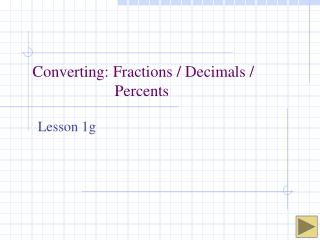DownloadDownload PresentationConverting: Fractions / Decimals / Percents

# Converting: Fractions / Decimals / Percents

Télécharger la présentation## Converting: Fractions / Decimals / Percents

- - - - - - - - - - - - - - - - - - - - - - - - - - - E N D - - - - - - - - - - - - - - - - - - - - - - - - - - -
##### Presentation Transcript

1. Decimals to Fractions

2. 1 2 How to Find the Decimal Value for a Fraction To do this - - divide the numerator by denominator. 1 divided by 2 equals .5 so that means the decimal value for 1/2 is .5

3. How to Find the Decimal Value for a Fraction Example 1: To do this - - divide the numerator by denominator. . 0 5 6 2 5 – 80 10 0 – 96 4 0 – 32 8 0 80 0 –

4. Fractions to Decimals Example 2: Divide the numerator by the denominator. .7 5 = .75 28 2 0 2 0 0

5. Your Turn .1 = = = .2 .3 .375 .38 .125 .5 1 2

6. Repeating Decimals For many fractions, the decimal equivalent repeats without end. Three dots means that the pattern continues without end. .032103210321 = .0321. . . It can also be indicated by a line over the number(s) that repeat. .037373737. . . = .037

7. Converting Decimals to Percents A percent (%) is a comparison of any number to 100. The word percent means“per hundred” You can use hundredth or percents to express the same number. 37 out of 100 squares are blue. 0.37 of the squares are blue. 37% of the squares are blue.

8. Decimals to percent (%) Percent means “per one hundred”. To convert a decimal to a percent you simply multiply by 100. When you multiply by 100, you simply move the decimal point over 2 places to the right! • 75 % . . . . • 75% .75 • 12.5% or 12 ½% • 12 5% . . . . .125

9. Converting Decimals to Percents • To convert a decimal to a percent when given a decimal such as 0.6, this is “6 tenths”. • But a percent is out of 100. • Therefore the only equivalent decimal to 0.6 with the hundredths place represented is 0.60, or in words 60 hundredths. • Then the percent is 60%.